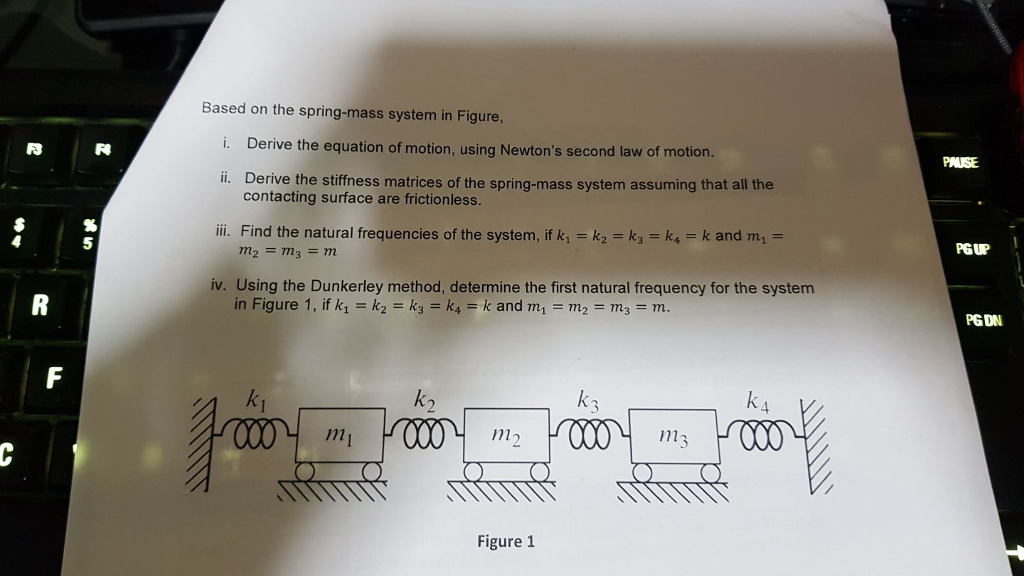### Create an Account

Home / Questions / Derive the stiffness matrices of the spring-mass system assuming that all the contacting s...

# Derive the stiffness matrices of the spring-mass system assuming that all the contacting surface are frictionless Image result for Derive the stiffness matrices of the spring mass system assuming that

Derive the stiffness matrices of the spring-mass system assuming that all the contacting surface are frictionlessJun 25 2020 View more View LessSubscribe To Get Solution